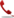+91-9818369374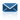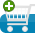Ask an Expert for Free Submit Your Assignment/Home work1 Live Tutoring Free Trial / 30 Min Sample Assignment / Home Work Make Payment Course Offered Resources
Quick Contact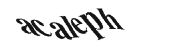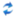# Question

The temperature g (t) at time t of a given point of a heated iron rod is given by

g (t) =,           where t > 0.

(a)     Find the interval where g¢ (t) > 0.

(4)

(b)     Find the interval where g² (t) > 0 and the interval where g² (t) < 0.

(5)

(c)     Find the value of t where the graph of g (t) has a point of inflexion.

(3)

(d)     Let t* be a value of t for which g¢ (t*) = 0 and g² (t*) < 0. Find t*.

(3)

(e)     Find the point where the normal to the graph of g (t) at the point
(t*, g (t*)) meets the t-axis.

(3)

(Total 18 marks)

Let f (x) = ln |x5 – 3x2|, –0.5 < x < 2, x ¹ a, x ¹ b; (a, b are values of x for which f (x) is not defined).

(a)     (i)      Sketch the graph of f (x), indicating on your sketch the number of zeros of f (x). Show also the position of any asymptotes.

(2)

(ii)     Find all the zeros of f (x), (that is, solve f (x) = 0).

(3)

(b)     Find the exact values of a and b.

(3)

(c)     Find f (x), and indicate clearly where f¢ (x) is not defined.

(3)

(d)     Find the exact value of the x-coordinate of the local maximum of f (x), for 0 < x < 1.5. (You may assume that there is no point of inflexion.)

(3)

(e)     Write down the definite integral that represents the area of the region enclosed by f (x) and the x-axis. (Do not evaluate the integral.)

AssignmentWork Payment (USD)
Content